# ICSE Class 8 Maths Selina Solutions for Chapter 17 Special Types of Quadrilaterals

A quadrilateral is a four-sided figure in 2-dimensional space made up of four line segments. The most common types of quadrilaterals are Squares and Rectangles. In the primary classes, we have studied the basic quadrilaterals. But in this Chapter, you will learn about special types of quadrilaterals. ICSE Class 8 maths Selina Solution Chapter 17 “Special types of Quadrilaterals” explains about parallelograms, rectangle, square, trapezium, and rhombus. The questions asked from this chapter is mostly related to the properties of different types of quadrilaterals.

The solutions of this chapter have been prepared by BYJU’S experts and students should practice these questions so that they can clear all their doubts. In your final exam paper, you’ll definitely find some of the questions asked from this chapter. Students can download the pdf format of ICSE Selina Solution Class 8 Maths “Special types of Quadrilaterals” solution from the link provided below.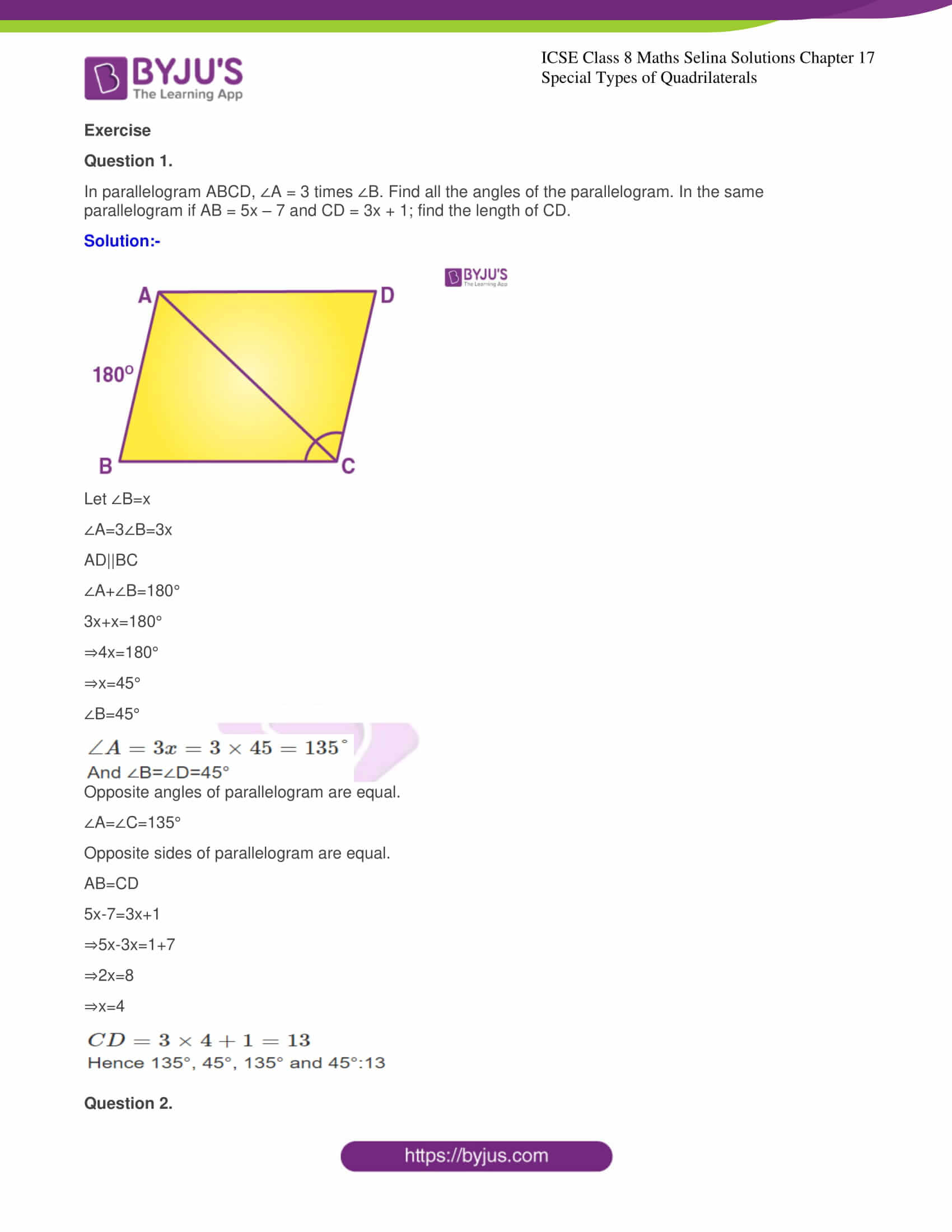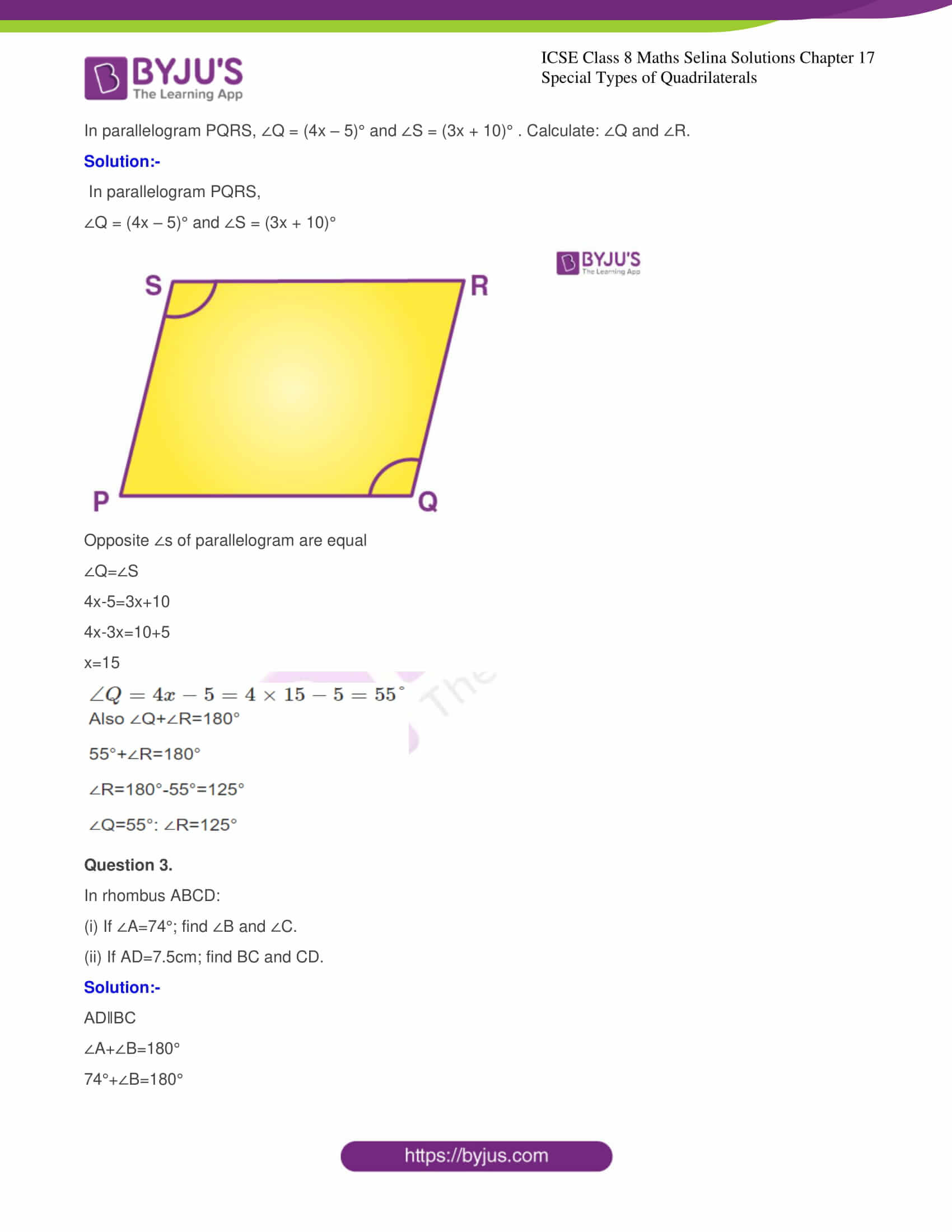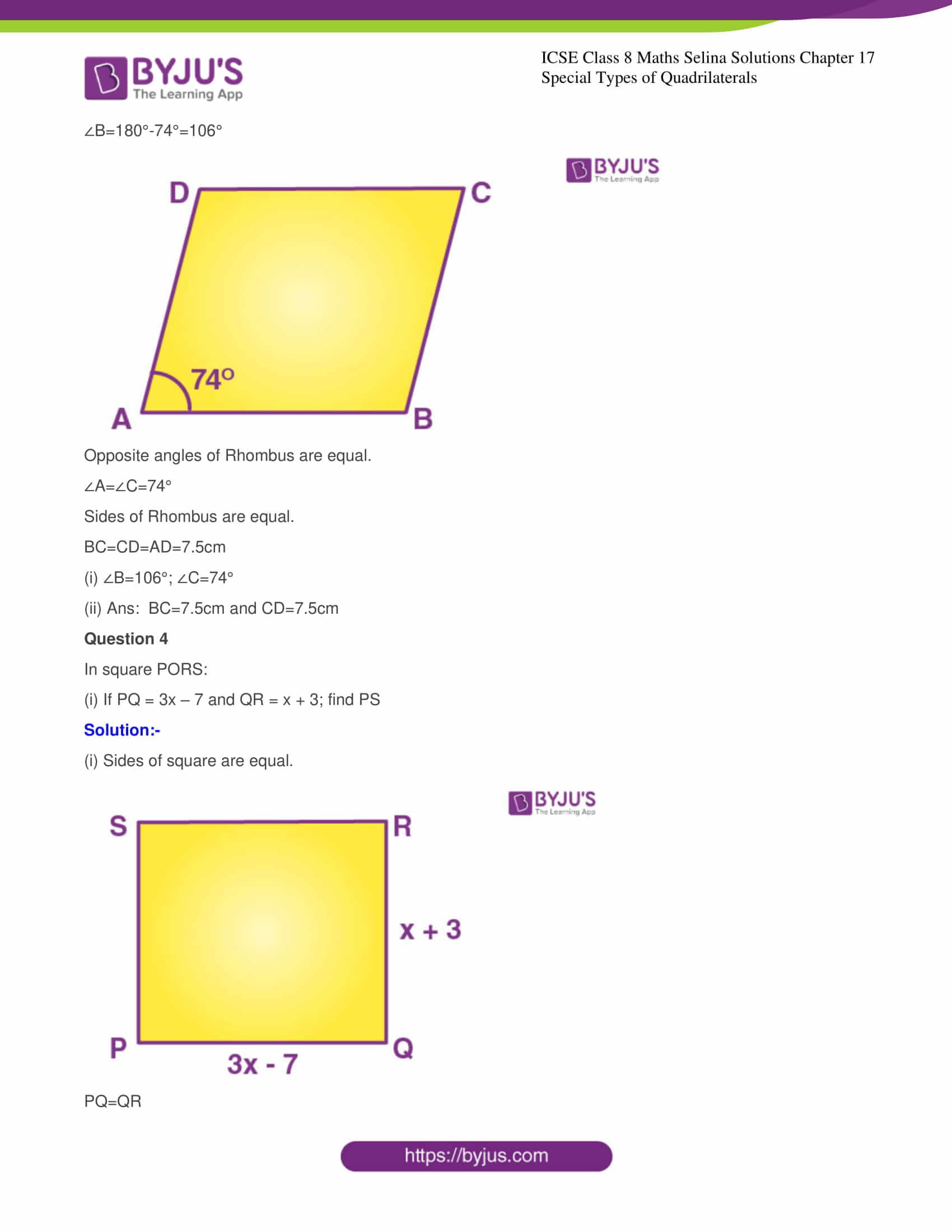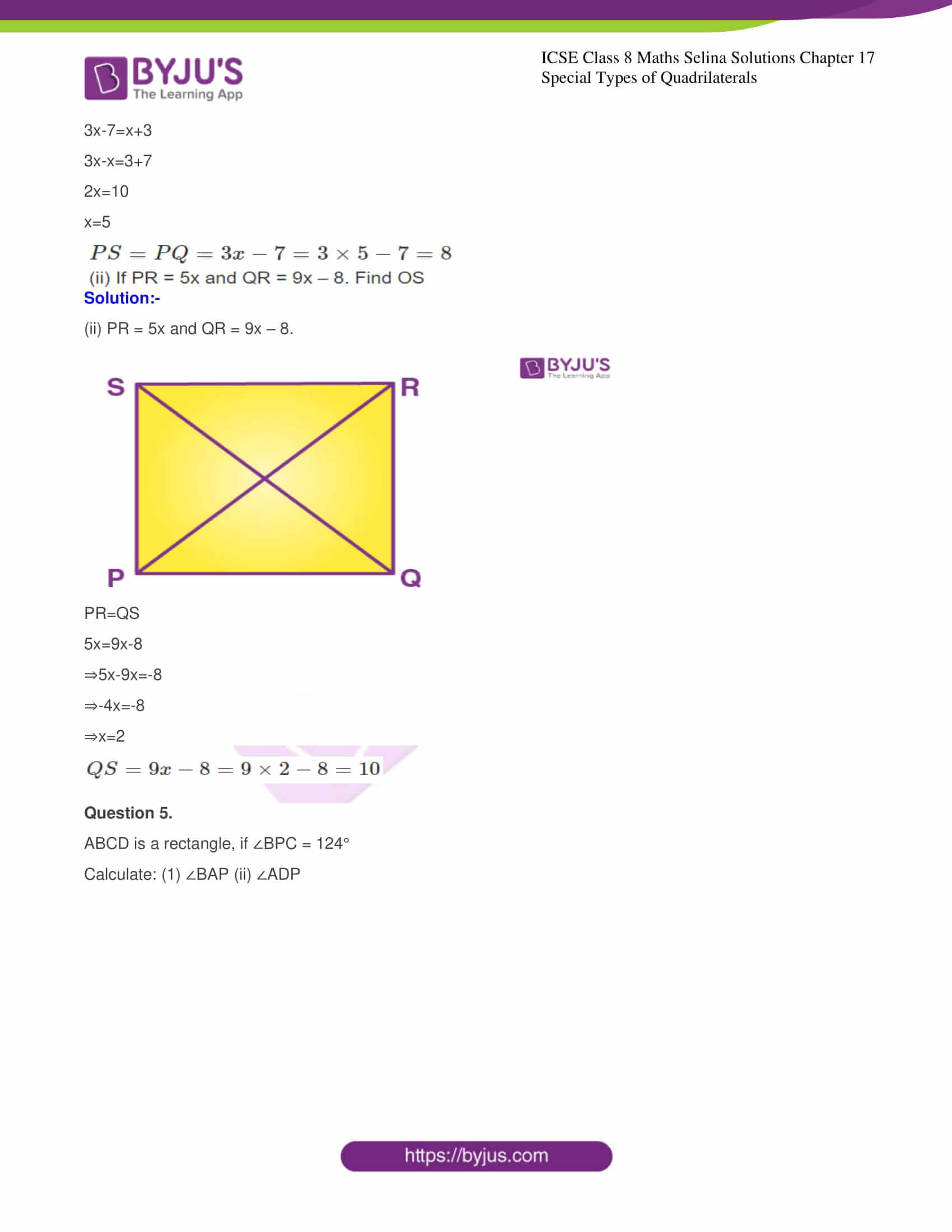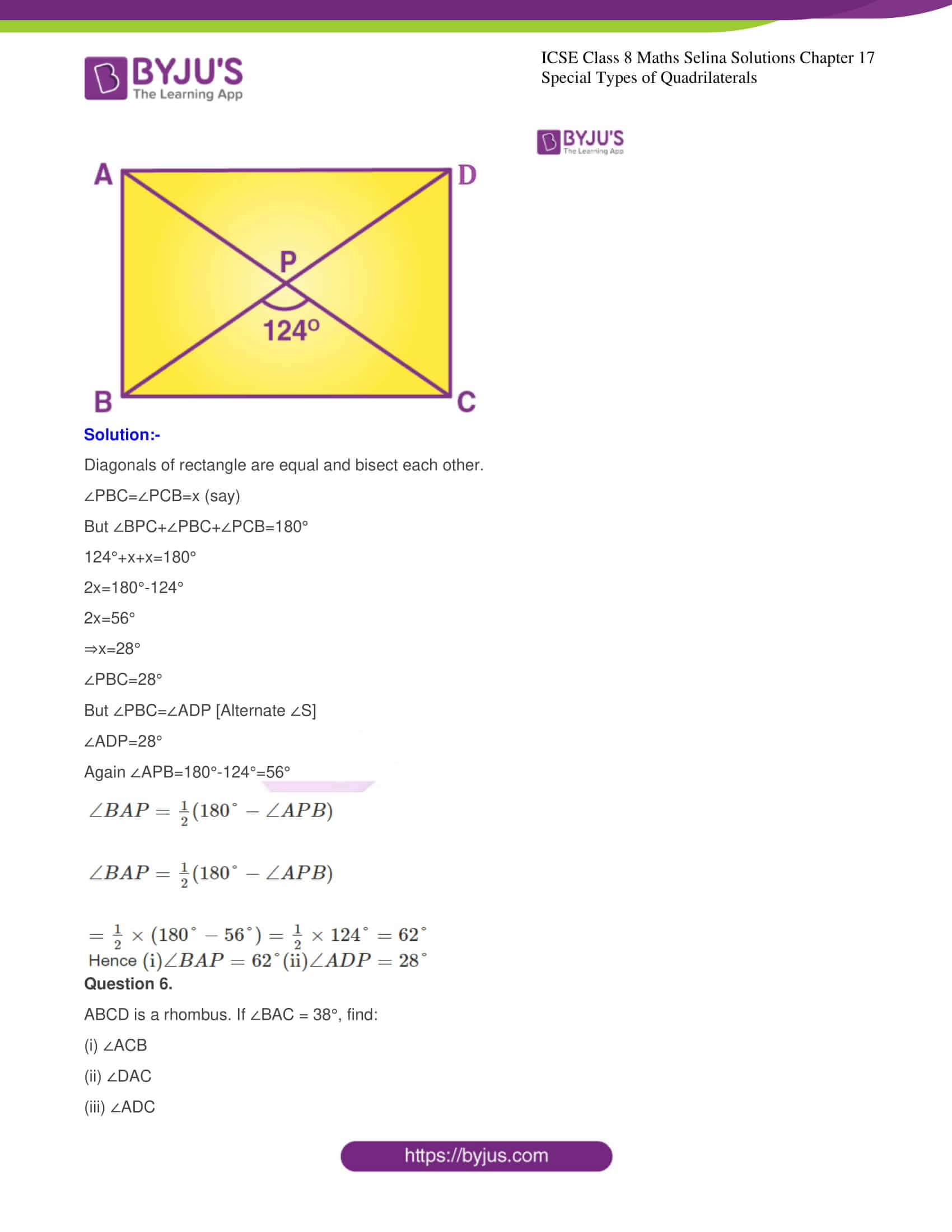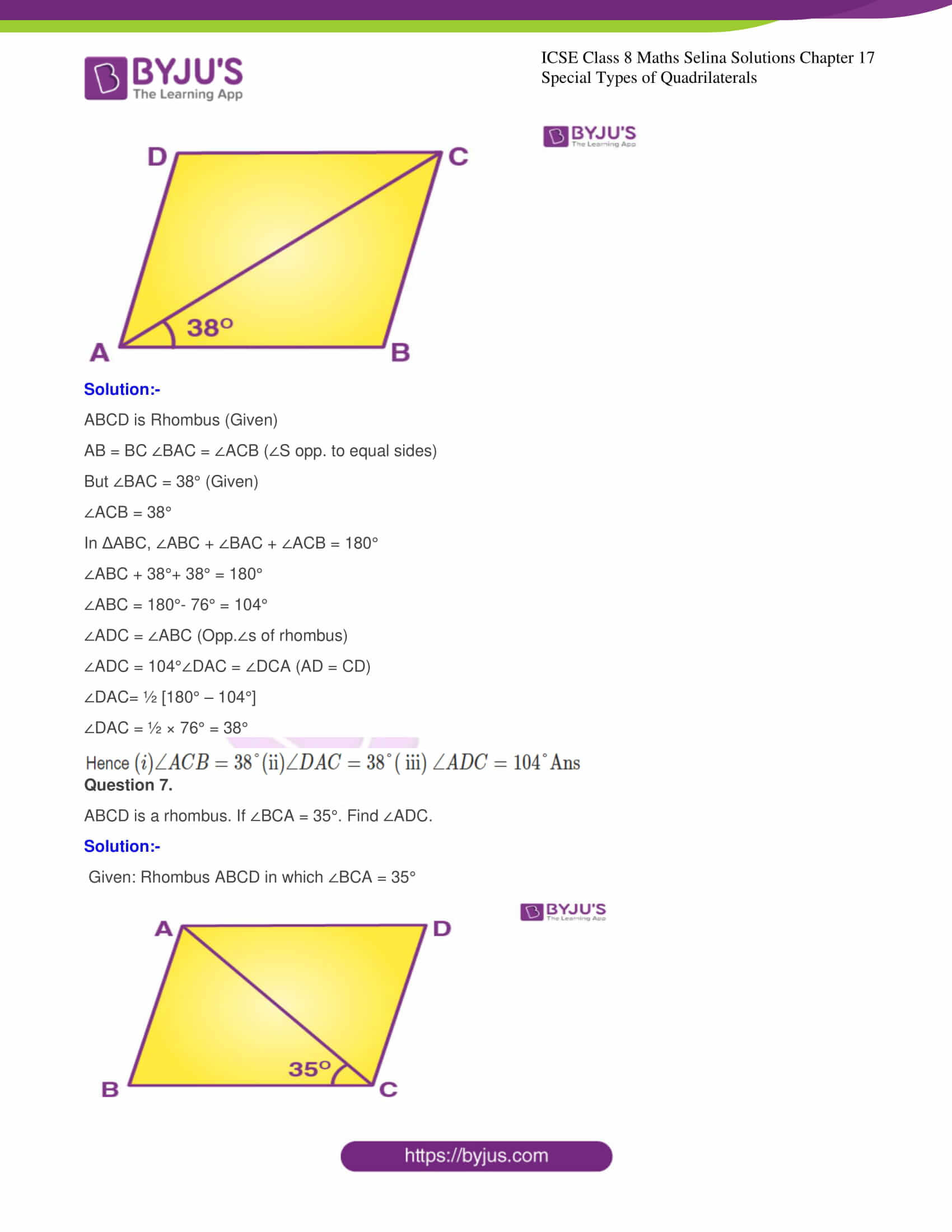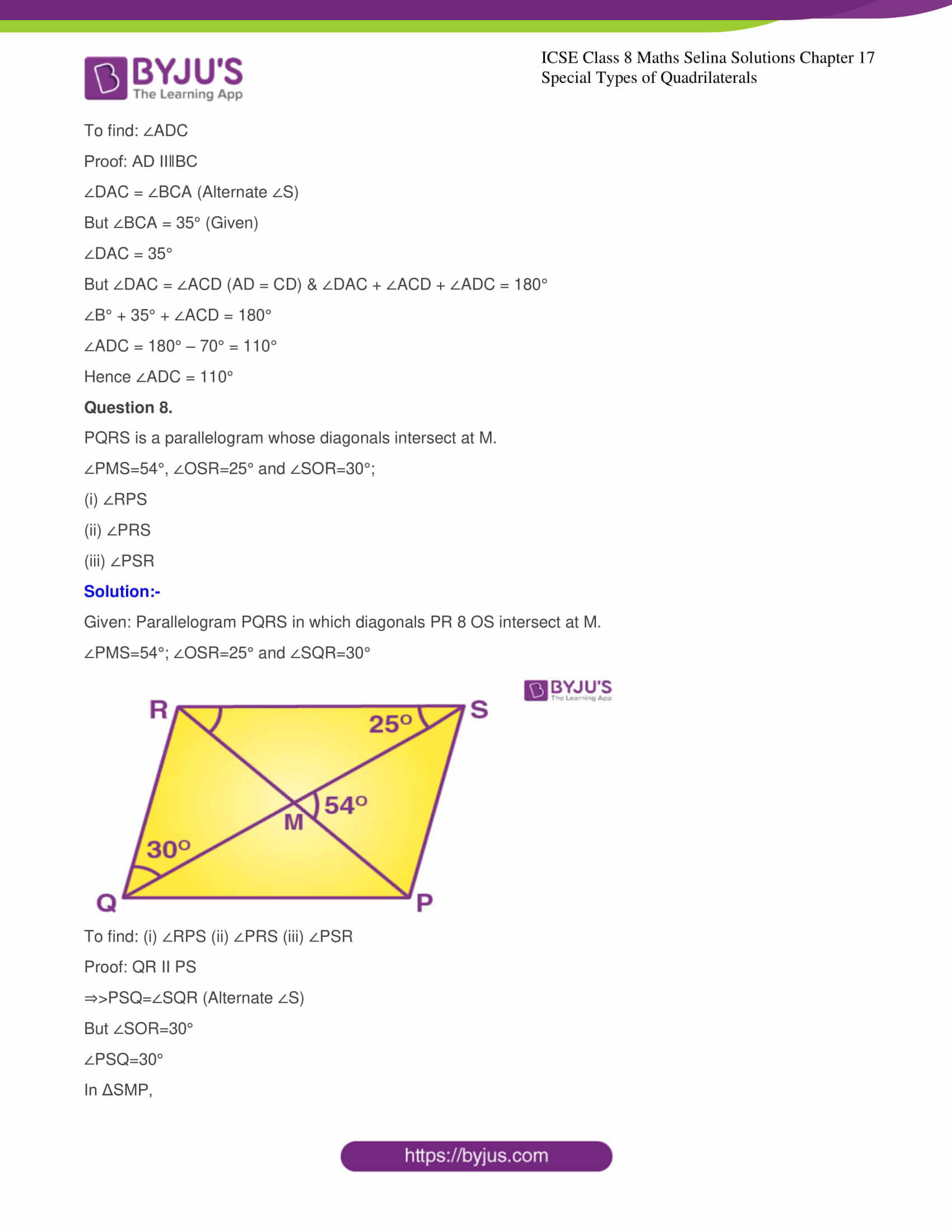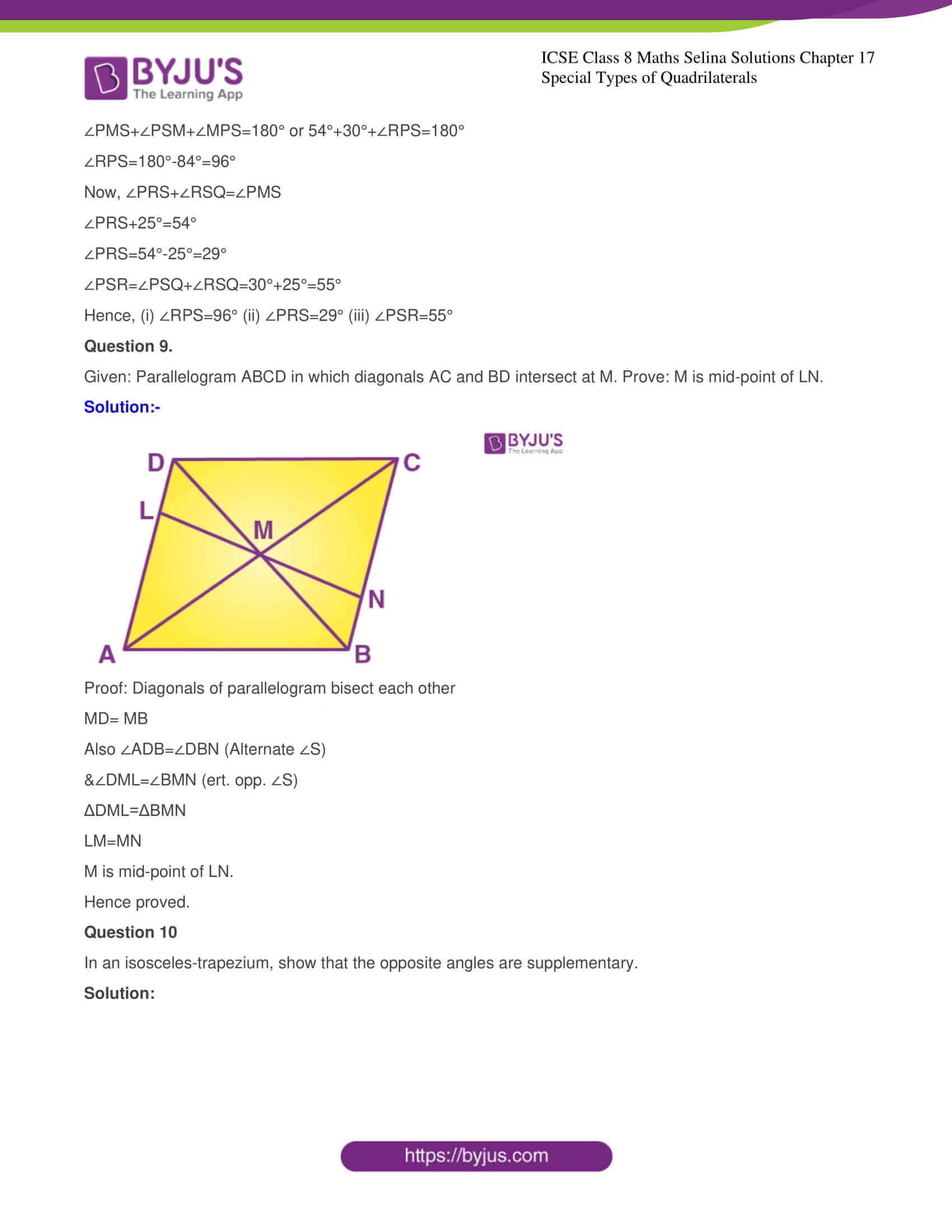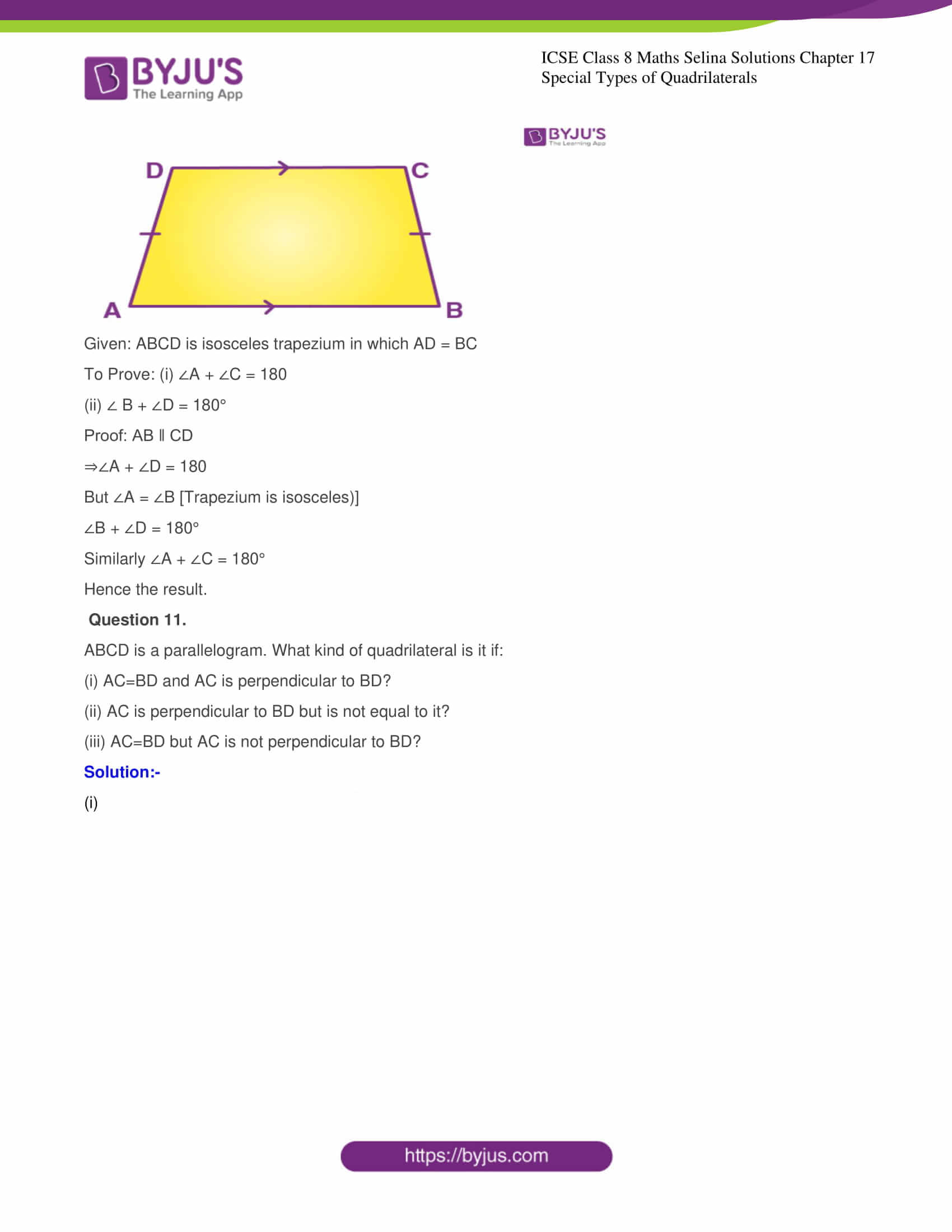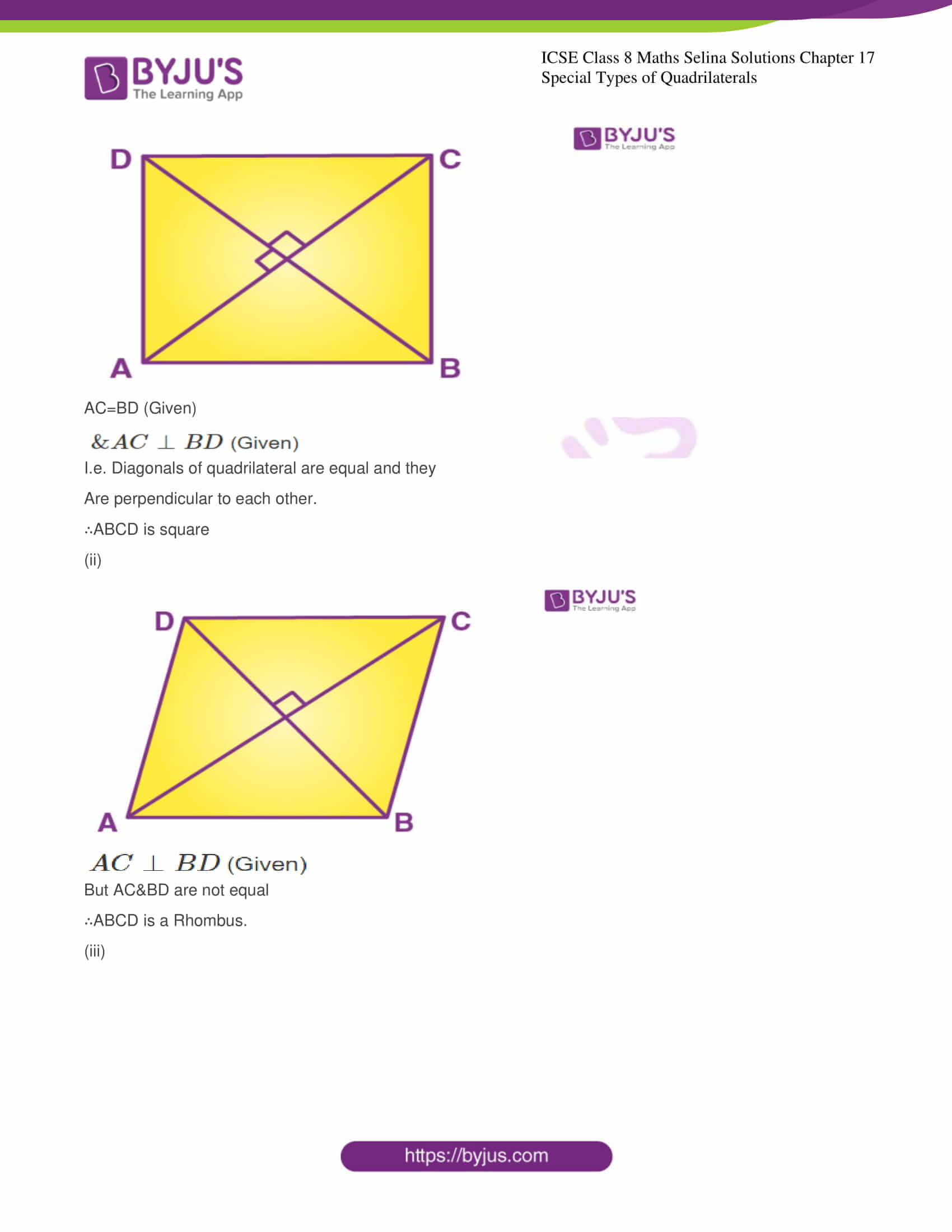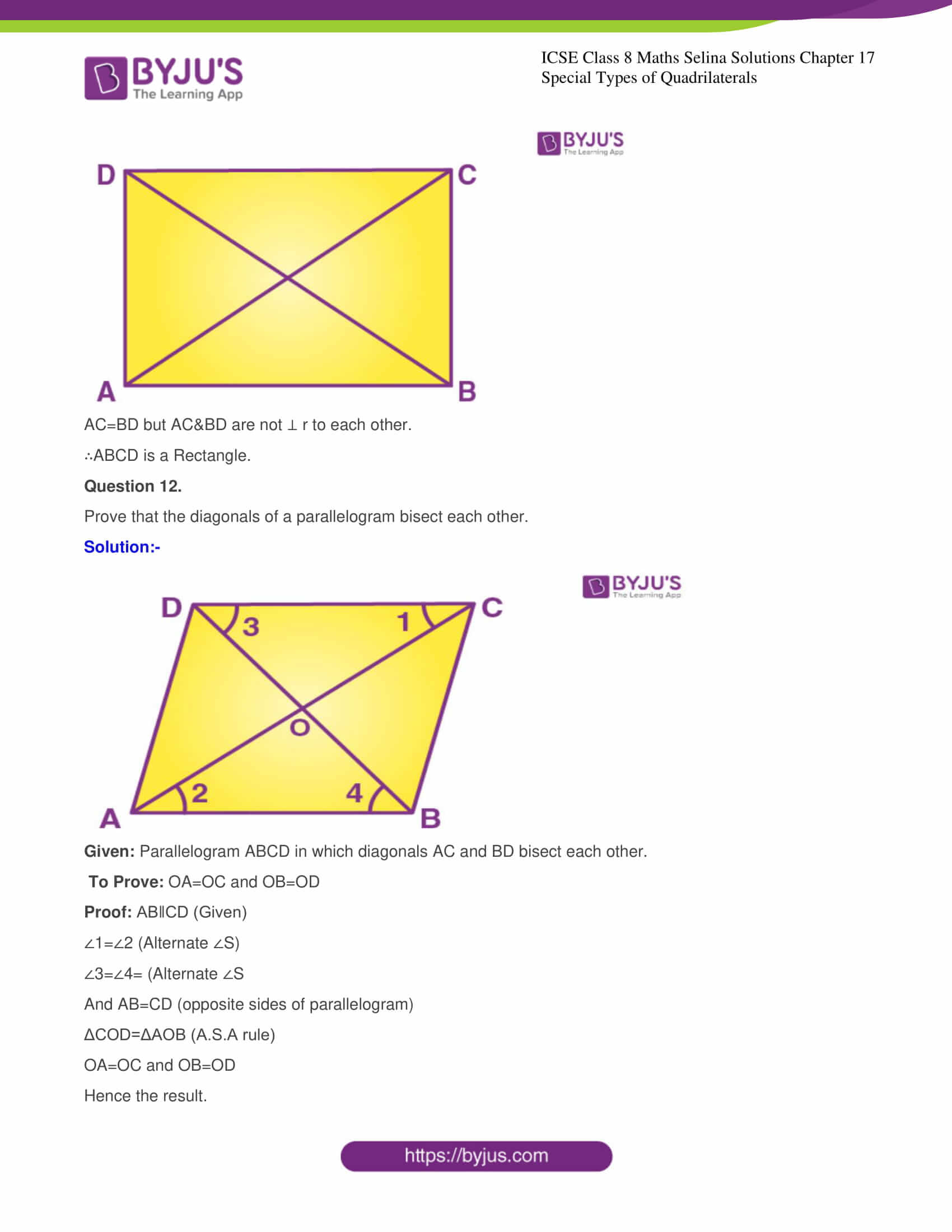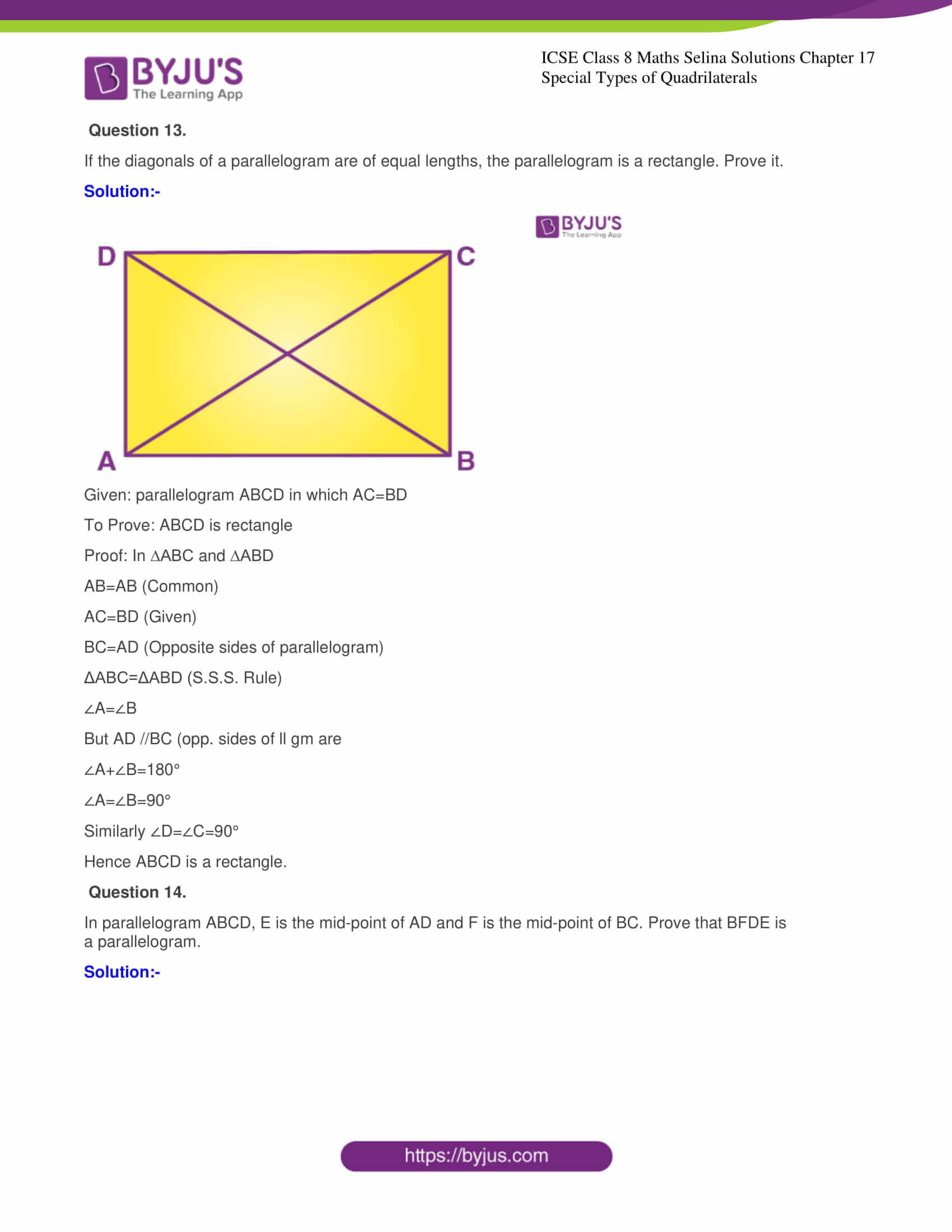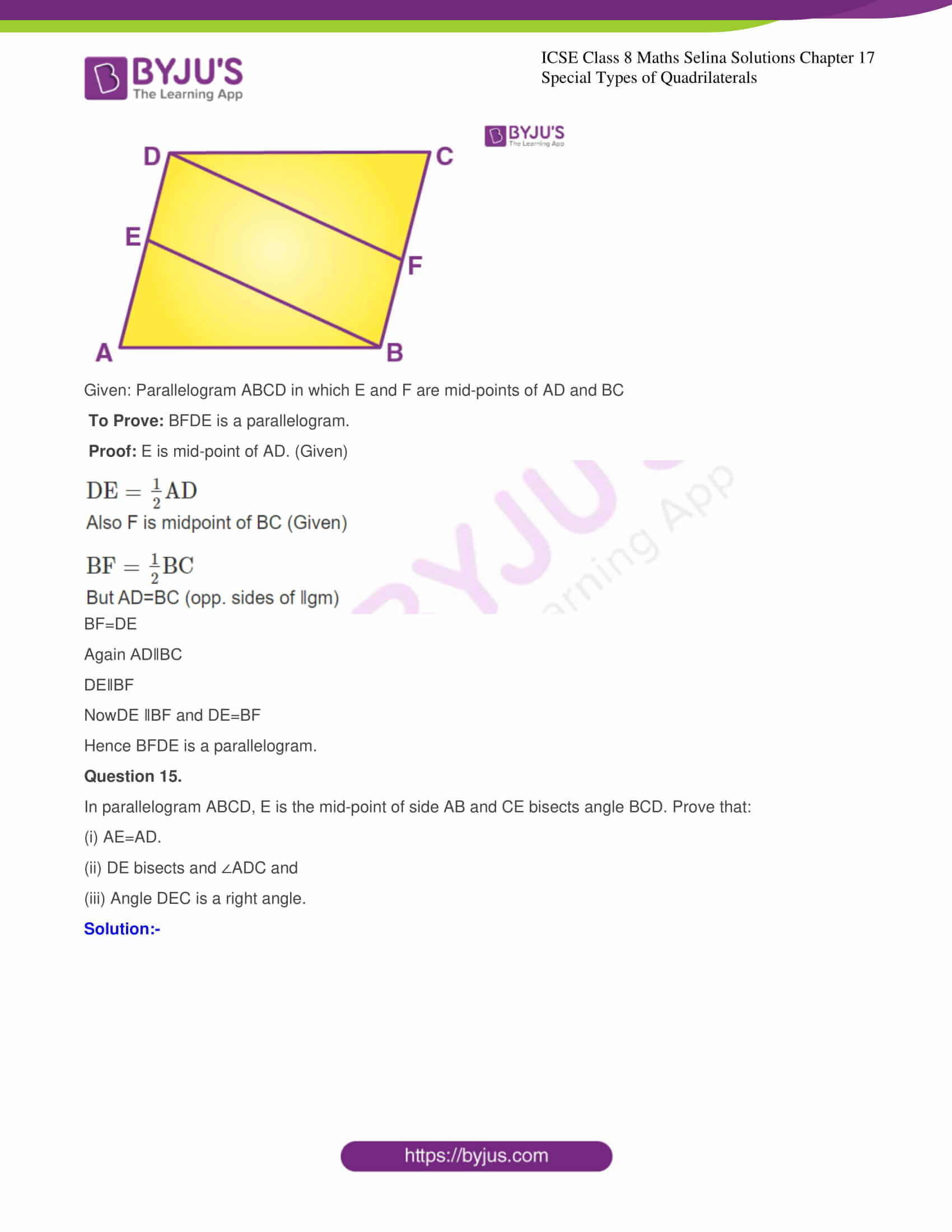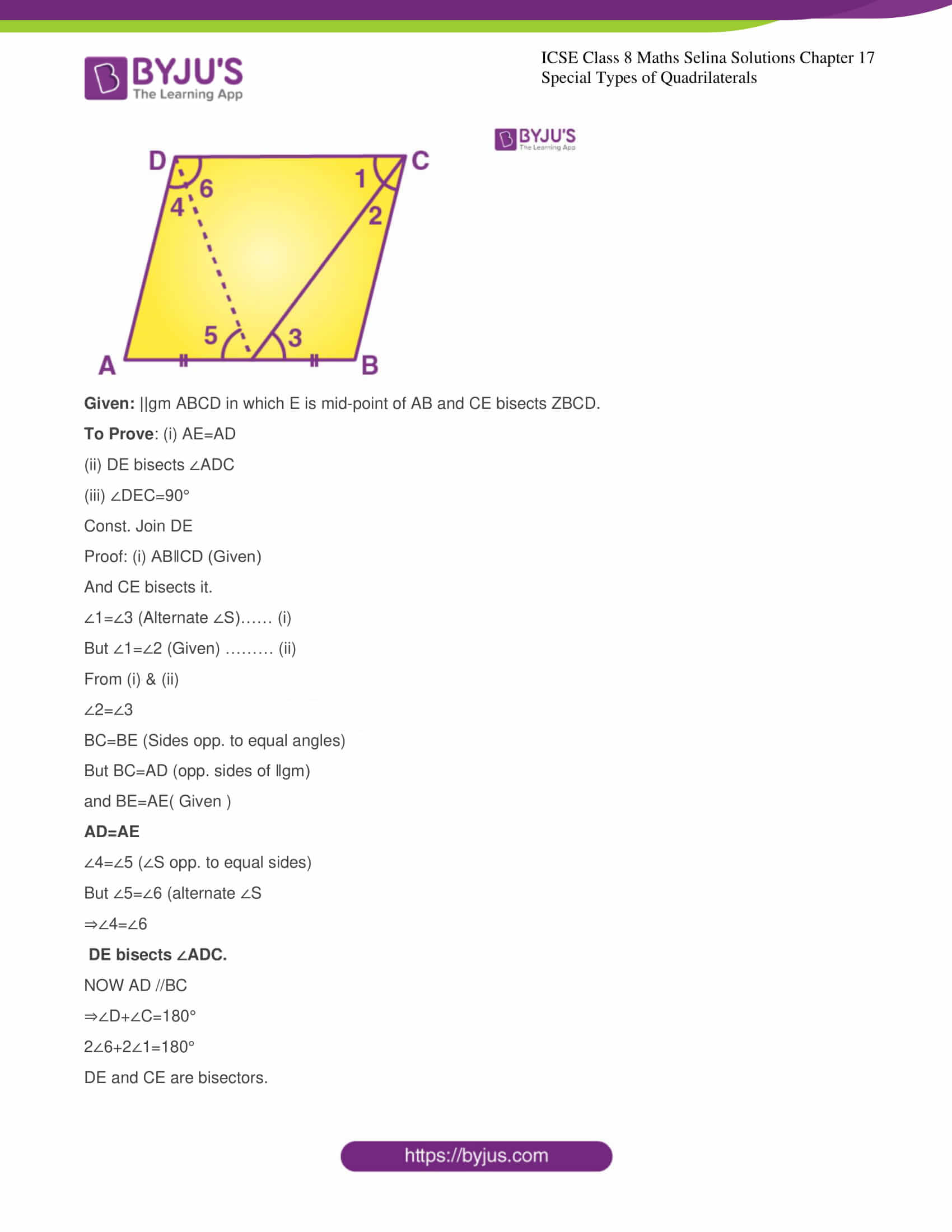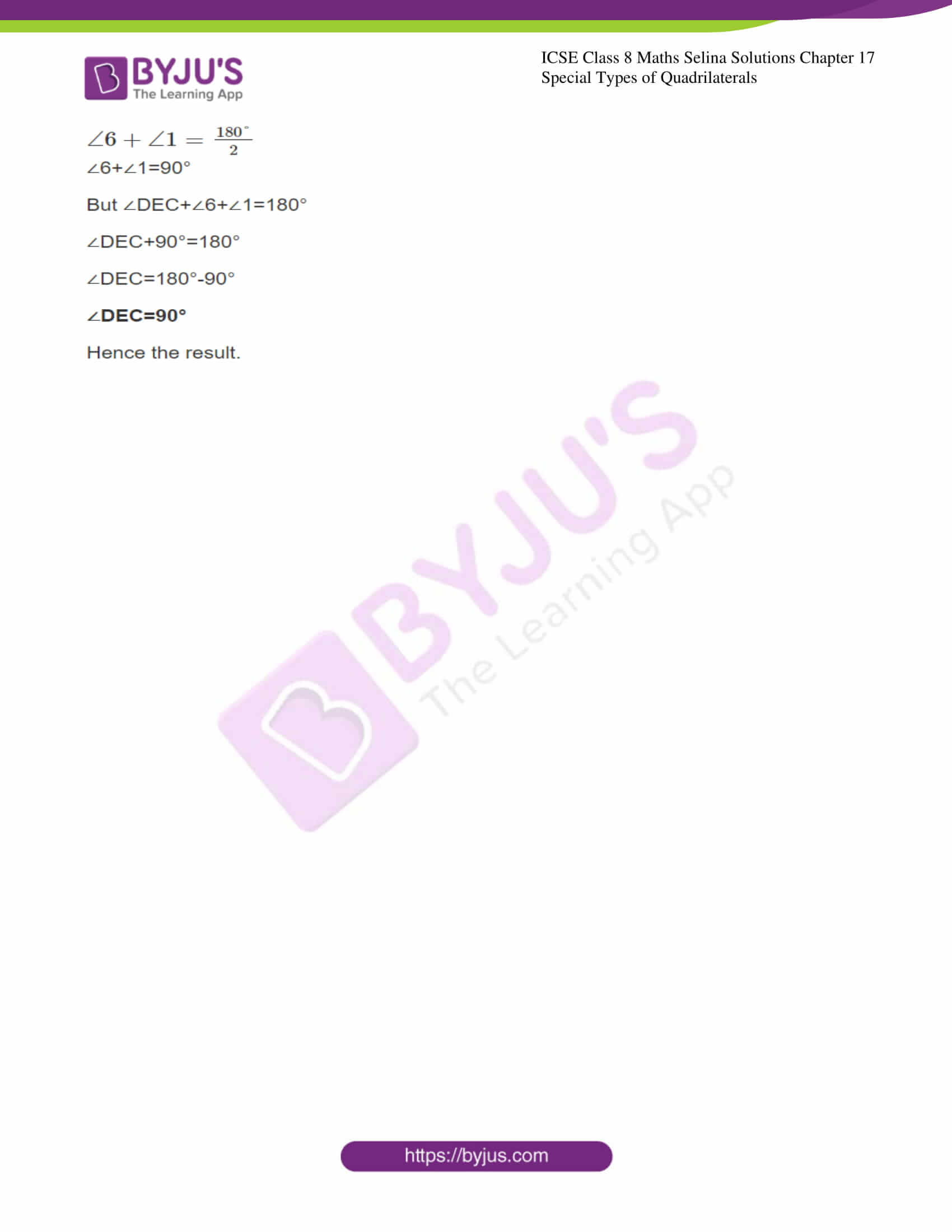Chapter 17 Special Types of Quadrilaterals consists of a total of 15 questions and the solutions of all these questions are provided below:

### CHAPTER 17 – SPECIAL TYPES OF QUADRILATERALS

Exercise

Question 1.

In parallelogram ABCD, ∠A = 3 times ∠B. Find all the angles of the parallelogram. In the same parallelogram if AB = 5x – 7 and CD = 3x + 1; find the length of CD.

Solution:-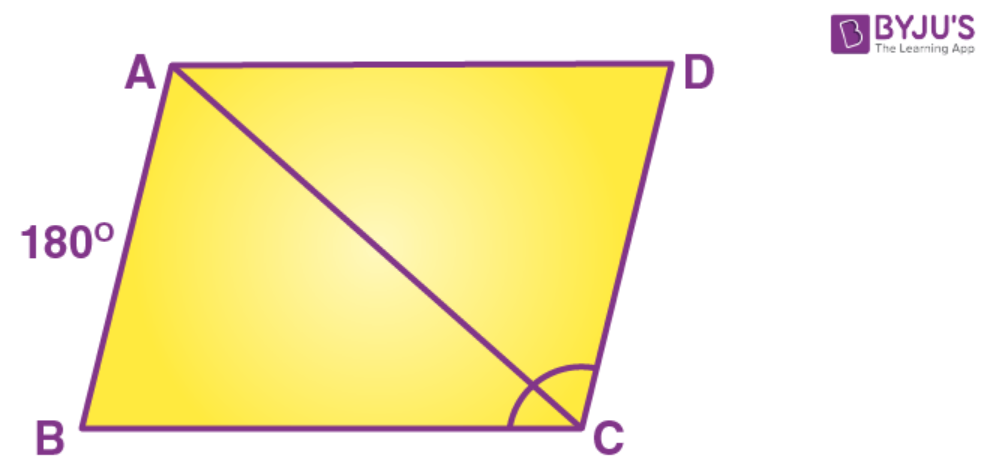Let ∠B=x

∠A=3∠B=3x

∠A+∠B=180°

3x+x=180°

⇒4x=180°

⇒x=45°

∠B=45°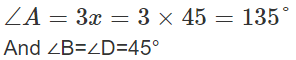Opposite angles of parallelogram are equal.

∠A=∠C=135°

Opposite sides of parallelogram are equal.

AB=CD

5x-7=3x+1

⇒5x-3x=1+7

⇒2x=8

⇒x=4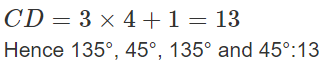Question 2.

In parallelogram PQRS, ∠Q = (4x – 5)° and ∠S = (3x + 10)° . Calculate: ∠Q and ∠R.

Solution:-

In parallelogram PQRS,

∠Q = (4x – 5)° and ∠S = (3x + 10)°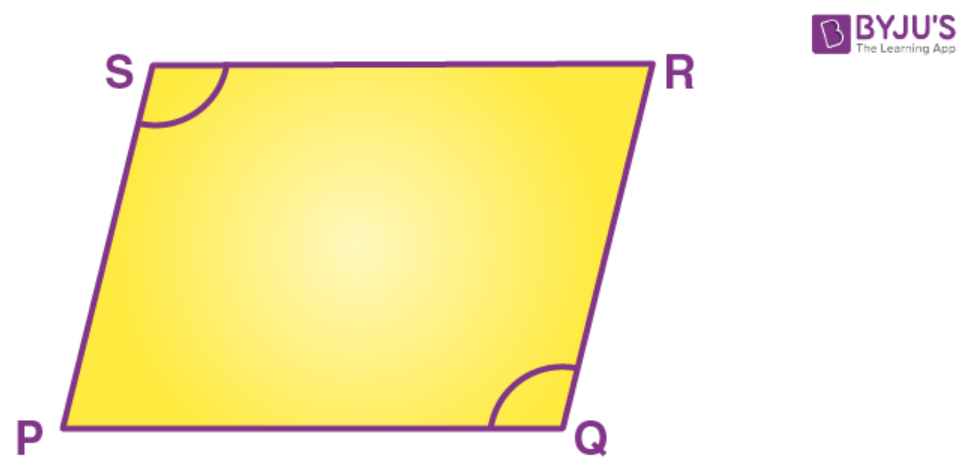Opposite ∠s of parallelogram are equal

∠Q=∠S

4x-5=3x+10

4x-3x=10+5

x=15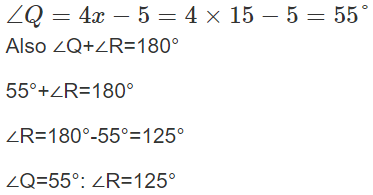Question 3.

In rhombus ABCD:

(i) If ∠A=74°; find ∠B and ∠C.

(ii) If AD=7.5cm; find BC and CD.

Solution:-

∠A+∠B=180°

74°+∠B=180°

∠B=180°-74°=106°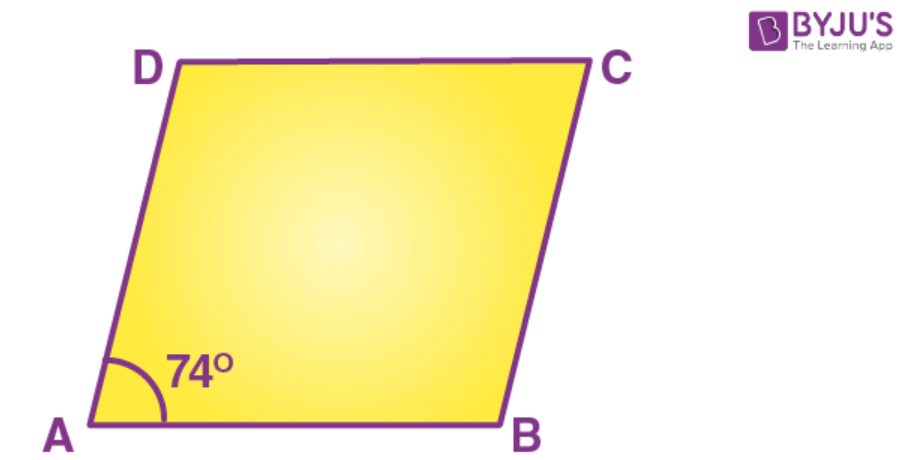Opposite angles of Rhombus are equal.

∠A=∠C=74°

Sides of Rhombus are equal.

(i) ∠B=106°; ∠C=74°

(ii) Ans:  BC=7.5cm and CD=7.5cm

Question 4

In square PORS:

(i) If PQ = 3x – 7 and QR = x + 3; find PS

Solution:-

(i) Sides of square are equal.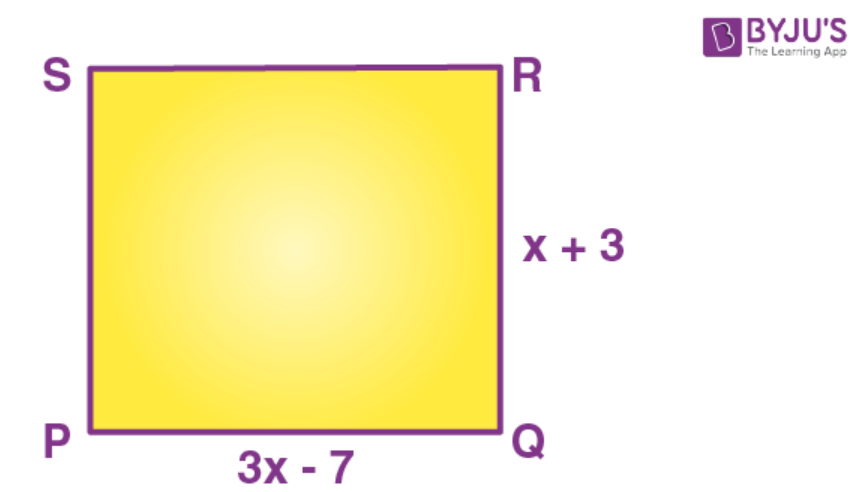PQ=QR

3x-7=x+3

3x-x=3+7

2x=10

x=5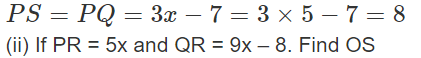Solution:-

(ii) PR = 5x and QR = 9x – 8.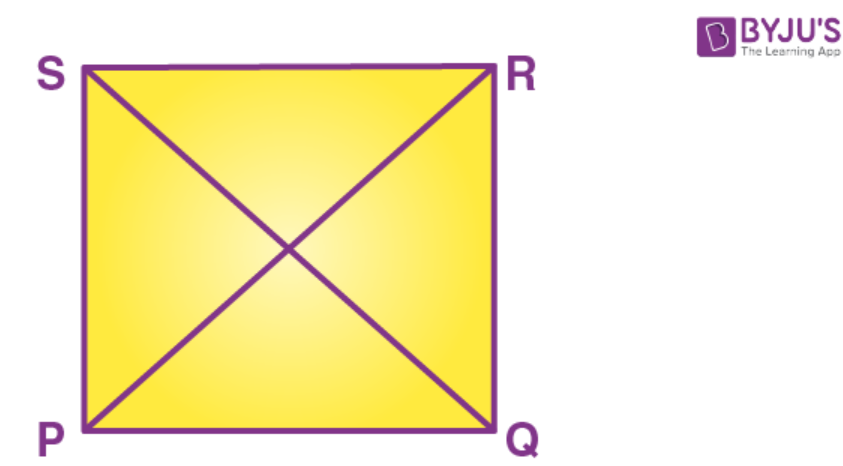PR=QS

5x=9x-8

⇒5x-9x=-8

⇒-4x=-8

⇒x=2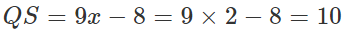Question 5.

ABCD is a rectangle, if ∠BPC = 124°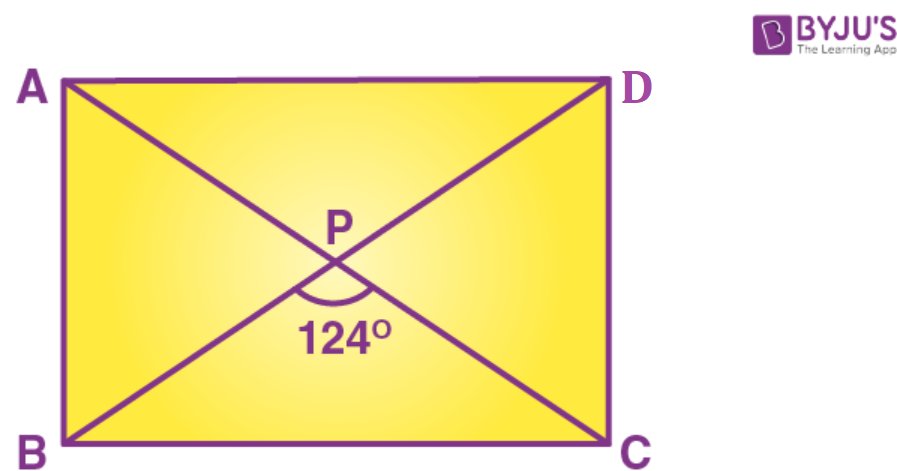Solution:-

Diagonals of rectangle are equal and bisect each other.

∠PBC=∠PCB=x (say)

But ∠BPC+∠PBC+∠PCB=180°

124°+x+x=180°

2x=180°-124°

2x=56°

⇒x=28°

∠PBC=28°

Again ∠APB=180°-124°=56°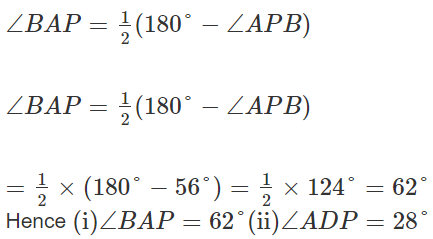Question 6.

ABCD is a rhombus. If ∠BAC = 38°, find:

(i) ∠ACB

(ii) ∠DAC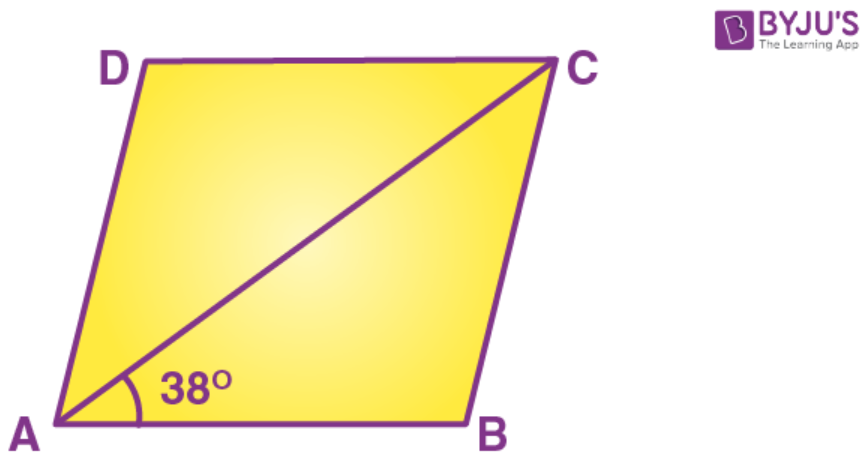Solution:-

ABCD is Rhombus (Given)

AB = BC ∠BAC = ∠ACB (∠S opp. to equal sides)

But ∠BAC = 38° (Given)

∠ACB = 38°

In ΔABC, ∠ABC + ∠BAC + ∠ACB = 180°

∠ABC + 38°+ 38° = 180°

∠ABC = 180°- 76° = 104°

∠ADC = ∠ABC (Opp.∠s of rhombus)

∠DAC= ½ [180° – 104°]

∠DAC = ½ × 76° = 38°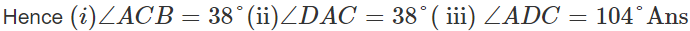Question 7.

ABCD is a rhombus. If ∠BCA = 35°. Find ∠ADC.

Solution:-

Given: Rhombus ABCD in which ∠BCA = 35°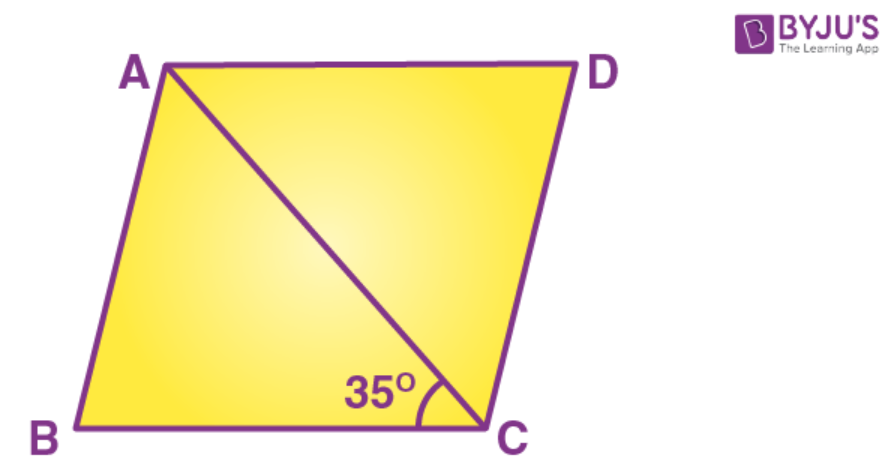∠DAC = ∠BCA (Alternate ∠S)

But ∠BCA = 35° (Given)

∠DAC = 35°

But ∠DAC = ∠ACD (AD = CD) & ∠DAC + ∠ACD + ∠ADC = 180°

∠B° + 35° + ∠ACD = 180°

∠ADC = 180° – 70° = 110°

Question 8.

PQRS is a parallelogram whose diagonals intersect at M.

∠PMS=54°, ∠OSR=25° and ∠SOR=30°;

(i) ∠RPS

(ii) ∠PRS

(iii) ∠PSR

Solution:-

Given: Parallelogram PQRS in which diagonals PR 8 OS intersect at M.

∠PMS=54°; ∠OSR=25° and ∠SQR=30°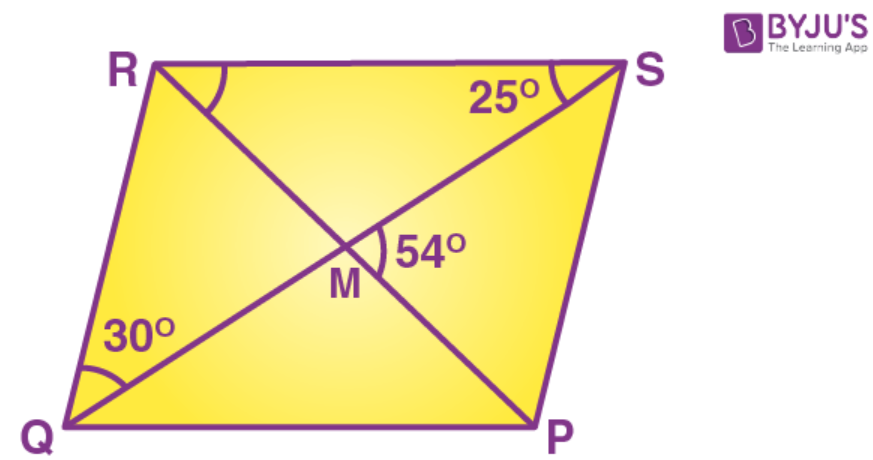To find: (i) ∠RPS (ii) ∠PRS (iii) ∠PSR

Proof: QR II PS

⇒>PSQ=∠SQR (Alternate ∠S)

But ∠SOR=30°

∠PSQ=30°

In ΔSMP,

∠PMS+∠PSM+∠MPS=180° or 54°+30°+∠RPS=180°

∠RPS=180°-84°=96°

Now, ∠PRS+∠RSQ=∠PMS

∠PRS+25°=54°

∠PRS=54°-25°=29°

∠PSR=∠PSQ+∠RSQ=30°+25°=55°

Hence, (i) ∠RPS=96° (ii) ∠PRS=29° (iii) ∠PSR=55°

Question 9.

Given: Parallelogram ABCD in which diagonals AC and BD intersect at M. Prove: M is mid-point of LN.

Solution:-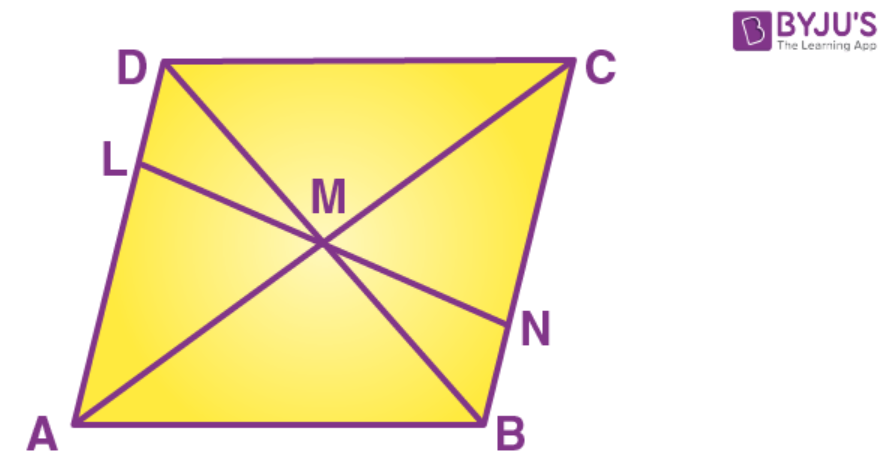Proof: Diagonals of parallelogram bisect each other

MD= MB

&∠DML=∠BMN (ert. opp. ∠S)

ΔDML=ΔBMN

LM=MN

M is mid-point of LN.

Hence proved.

Question 10

In an isosceles-trapezium, show that the opposite angles are supplementary.

Solution: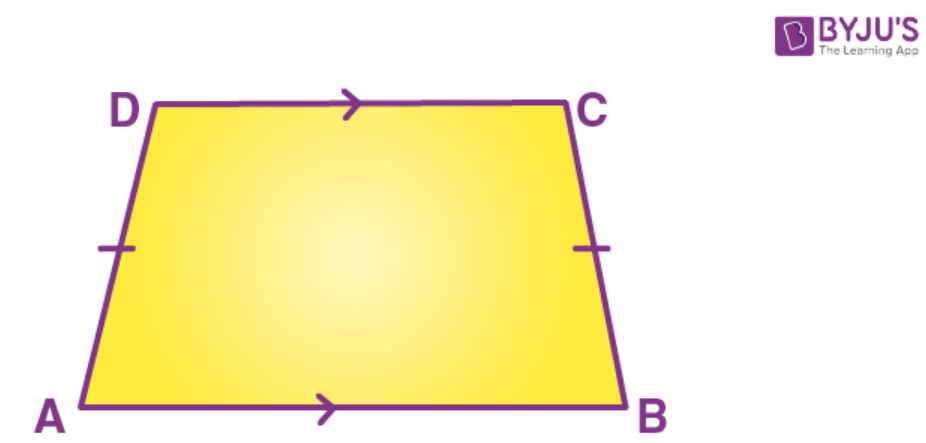Given: ABCD is isosceles trapezium in which AD = BC

To Prove: (i) ∠A + ∠C = 180

(ii) ∠ B + ∠D = 180°

Proof: AB ‖ CD

⇒∠A + ∠D = 180

But ∠A = ∠B [Trapezium is isosceles)]

∠B + ∠D = 180°

Similarly ∠A + ∠C = 180°

Hence the result.

Question 11.

ABCD is a parallelogram. What kind of quadrilateral is it if:

(i) AC=BD and AC is perpendicular to BD?

(ii) AC is perpendicular to BD but is not equal to it?

(iii) AC=BD but AC is not perpendicular to BD?

Solution:-

(i)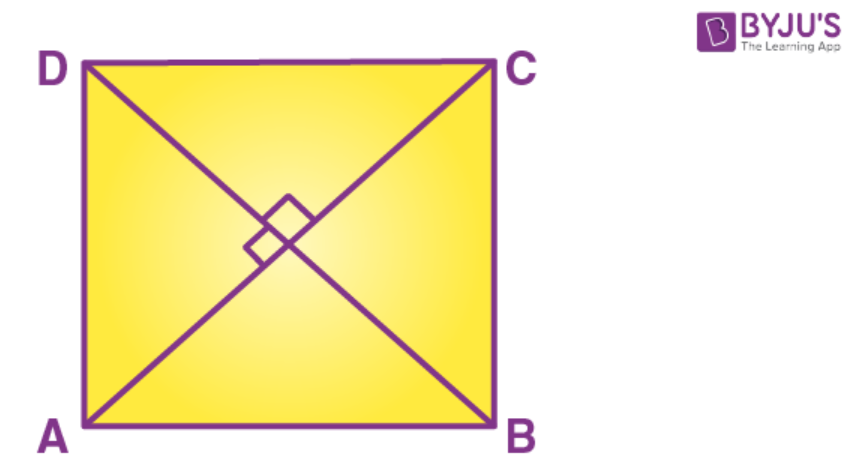AC=BD (Given)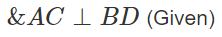I.e. Diagonals of quadrilateral are equal and they

Are perpendicular to each other.

∴ABCD is square

(ii)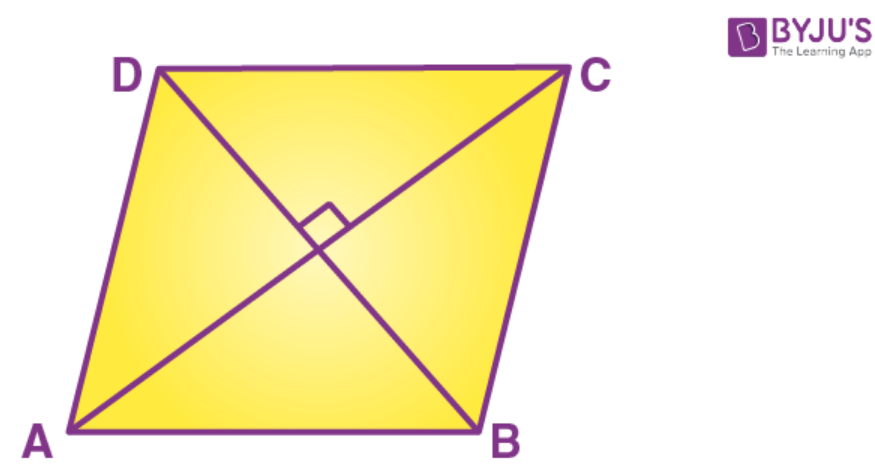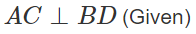But AC&BD are not equal

∴ABCD is a Rhombus.

(iii)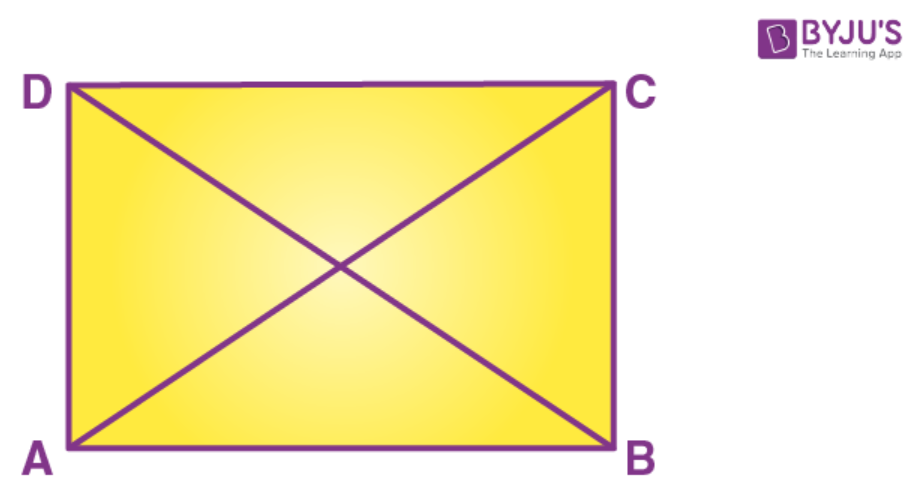AC=BD but AC&BD are not ⊥ r to each other.

∴ABCD is a Rectangle.

Question 12.

Prove that the diagonals of a parallelogram bisect each other.

Solution:-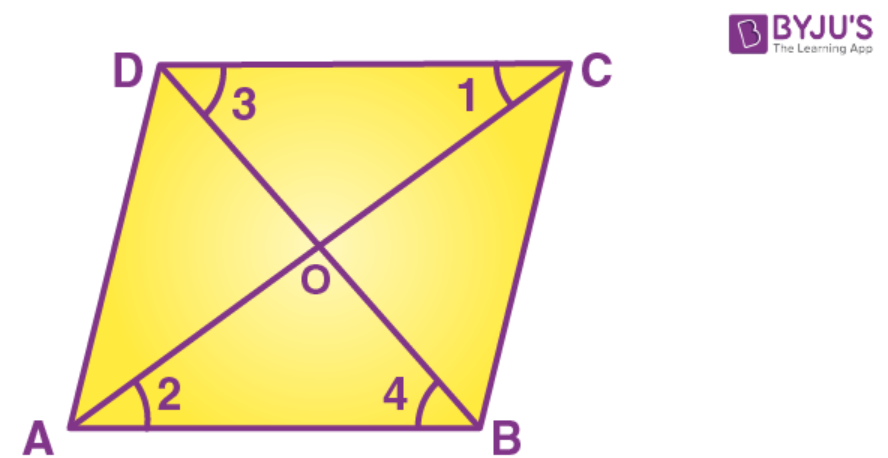Given: Parallelogram ABCD in which diagonals AC and BD bisect each other.

To Prove: OA=OC and OB=OD

Proof: AB‖CD (Given)

∠1=∠2 (Alternate ∠S)

∠3=∠4= (Alternate ∠S

And AB=CD (opposite sides of parallelogram)

ΔCOD=ΔAOB (A.S.A rule)

OA=OC and OB=OD

Hence the result.

Question 13.

If the diagonals of a parallelogram are of equal lengths, the parallelogram is a rectangle. Prove it.

Solution:-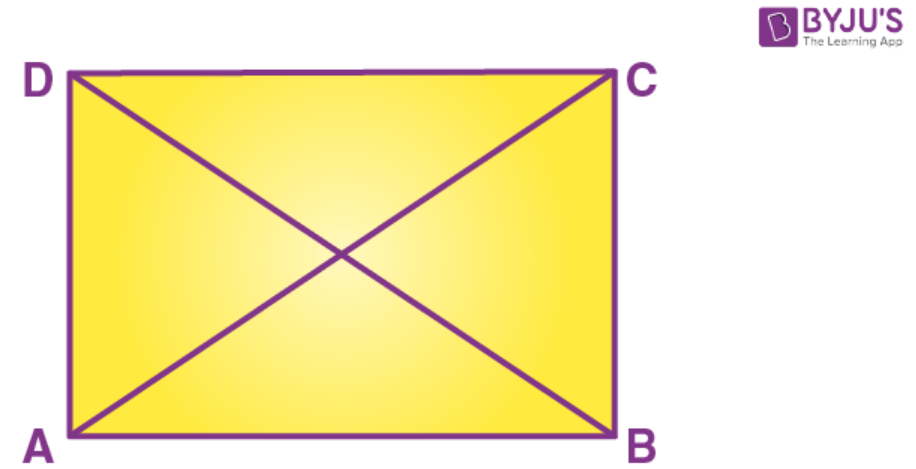Given: parallelogram ABCD in which AC=BD

To Prove: ABCD is rectangle

Proof: In ∆ABC and ∆ABD

AB=AB (Common)

AC=BD (Given)

ΔABC=ΔABD (S.S.S. Rule)

∠A=∠B

But AD //BC (opp. sides of ll gm are

∠A+∠B=180°

∠A=∠B=90°

Similarly ∠D=∠C=90°

Hence ABCD is a rectangle.

Question 14.

In parallelogram ABCD, E is the mid-point of AD and F is the mid-point of BC. Prove that BFDE is a parallelogram.

Solution:-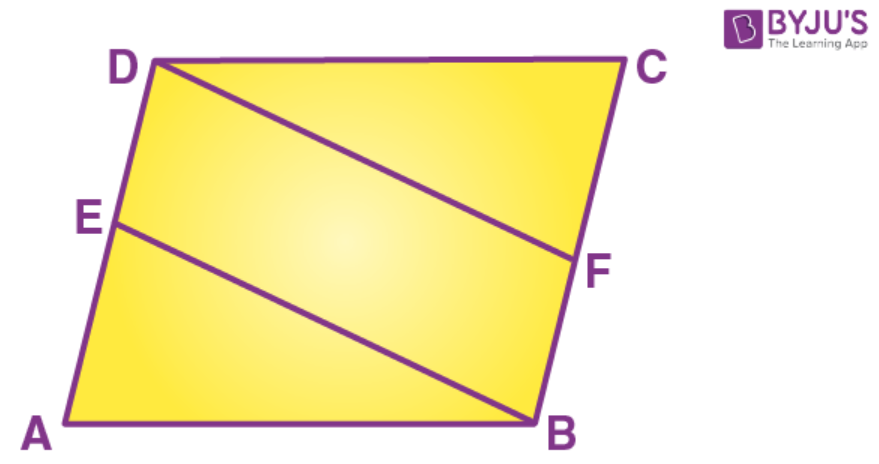Given: Parallelogram ABCD in which E and F are mid-points of AD and BC

To Prove: BFDE is a parallelogram.

Proof: E is mid-point of AD. (Given)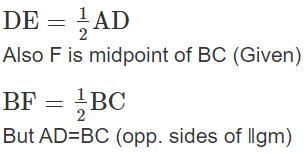BF=DE

DE‖BF

NowDE ‖BF and DE=BF

Hence BFDE is a parallelogram.

Question 15.

In parallelogram ABCD, E is the mid-point of side AB and CE bisects angle BCD. Prove that:

(ii) DE bisects and ∠ADC and

(iii) Angle DEC is a right angle.

Solution:-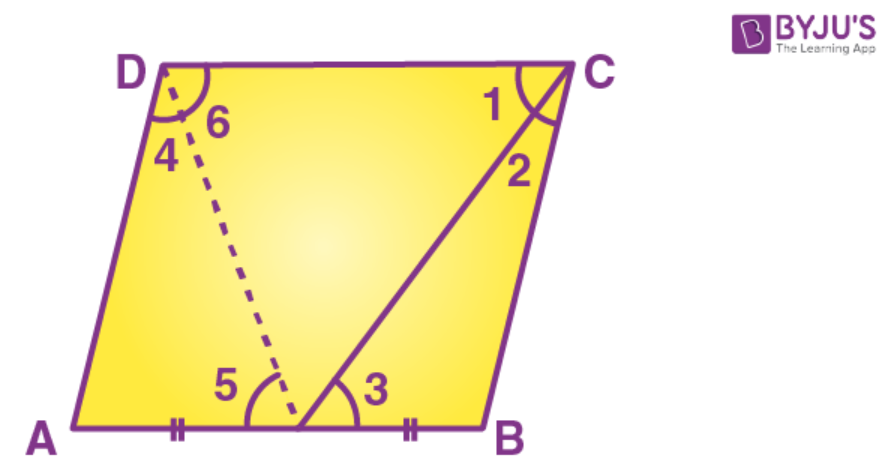Given: ||gm ABCD in which E is mid-point of AB and CE bisects ZBCD.

(iii) ∠DEC=90°

Const. Join DE

Proof: (i) AB‖CD (Given)

And CE bisects it.

∠1=∠3 (Alternate ∠S)…… (i)

But ∠1=∠2 (Given) ……… (ii)

From (i) & (ii)

∠2=∠3

BC=BE (Sides opp. to equal angles)

But BC=AD (opp. sides of ‖gm)

and BE=AE( Given )

∠4=∠5 (∠S opp. to equal sides)

But ∠5=∠6 (alternate ∠S

⇒∠4=∠6

⇒∠D+∠C=180°

2∠6+2∠1=180°

DE and CE are bisectors.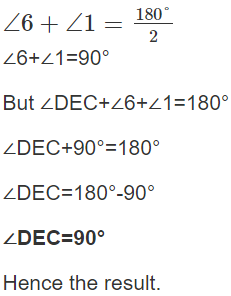### ICSE Class 8 Maths Selina Solutions Chapter 17 – Special Types of Quadrilaterals

In the earlier chapter, you have studied about understanding of shapes, theorems, etc. In Chapter 17 Special types of Quadrilaterals, you get to know about different types of quadrilaterals, its properties, formulas to solve the problems, etc. The concepts were explained beautifully in a detailed manner along with suitable examples wherever it’s needed. Also, the solutions provided here is from the exercises mentioned in the chapter. Students should solve these questions and if they have any doubt they can refer to the solutions.

Click on ICSE Class 8 Selina Solutions to access the answers of Physics, Chemistry and Biology subjects provided at one place. To access interactive Maths and Science Videos download BYJU’S App and subscribe to YouTube Channel.